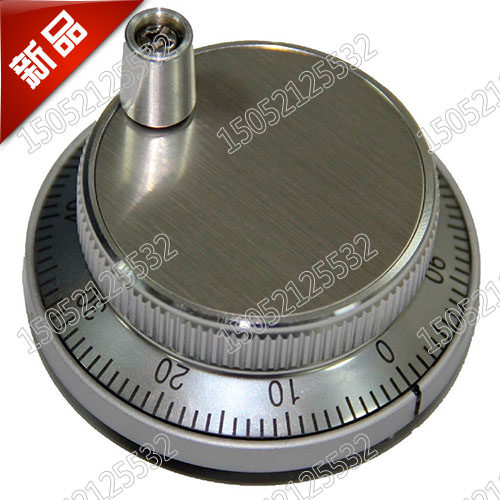# rotary encoder strange behavior (too many datas)

Posted on
• Hello,i'm playing with a rotary encoder a big MYSM60-100PPRR-5L with the example given
but the console is populated with too many answer for 1 step.

``````function onInit() {
var step = 0;
require("Encoder").connect(A5,A8, (dir) => {
print(dir);
if (dir > 0) {
//allume la led 20ms
digitalPulse(LED1, 1, 20);
} else {
digitalPulse(LED2, 1, 20);
}
});
}
``````

console return for 1step backward

``````-1
-1
-1
-1
-1
1
>
``````

and

``````1
1
1
1
>
``````

for 1 step forward...

• It matchs 4 time for 1 step

• i've corrected my pb with an ugly trick but it works as i need.
it sends through the serial :
`1` for clockwise and pulse Green led
`-1` for conterclockwise and pulse Red led

``````function onInit() {
var step = 0;
var final = 0;
require("Encoder").connect(A5, A8, (temp) => {
step += temp;
if (Math.floor(step / 4) == step / 4) {
(step > 0) ? dir = 1: dir = -1;
step = 0;
(dir > 0) ?digitalPulse(LED1, 1, 20):digitalPulse(LED2, 1, 20);
print(dir);
}
});
}
``````
• no more answer than mine... okay it's not really interesting, nevermind... there is a glitch in the lib...

and my attempt is not 100% efficient.
if I turn the encoder quickly, the algorithm slips, and the number advances slowly with a step backwards from time to time...

a better idea than mine?

regards

éric

• Sorry - I thought from the above that you may have sorted it... Espruino does actually include the ability to monitor a pin in a setWatch, so that may help you:

``````var value = 0;
setWatch(function(e) {
if (e.data) value++ else value--;
print(value);
}, A5, {data:A8, repeat:true});
``````

I notice you're doing a `print` and `digitalPulse`. Is it possible that turning the knob quick is creating so many events that the functions can't be called quick enough and so it loses track?

• Thanks Gordon
i've commented the digitalPulse and print to minimize the interference...
i send through HID

é.

• Hello @gordon
what is suppose to do?
`e.data` is always `false` when it fire. So, `value` stepdown what ever i turn clockwise or counterclockwise...

regards

é.

• That is strange... Do the pins need pullups?

Maybe swap `A5` and `A8` in the code and see if that helps. Also maybe you needed `edge:'falling'` in the watch.

Usually in an encoder, when one pin changes state, the state of the other pin determines the angle. But in this case I guess because we were reacting on both edges of A5, we'd just end up adding and subtracting from `value`

• OK thank you @Gordon

the final solution was the pull_up

``````function onInit() {
var value = 0;
pinMode(A8, 'input_pullup');
pinMode(A5, 'input_pullup');
setWatch(function(e) {
if (e.data) value++ else value--;
print(value);
}, A5, {data:A8, repeat:true, edge:'falling'});
}
``````

the value increases and decreases according to the rotation.

é.

•Post a reply